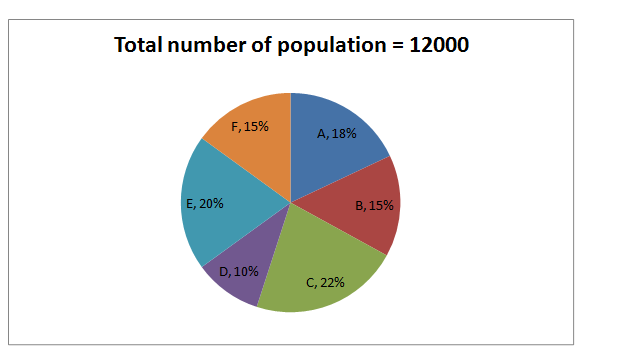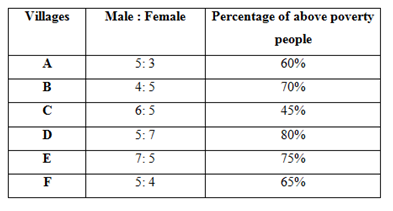# SBI Clerk Mains Quantitative Aptitude (Day-33)

Dear Aspirants, Our IBPS Guide team is providing new series of Quantitative Aptitude Questions for SBI Clerk Mains 2020 so the aspirants can practice it on a daily basis. These questions are framed by our skilled experts after understanding your needs thoroughly. Aspirants can practice these new series questions daily to familiarize with the exact exam pattern and make your preparation effective.

Start Quiz

Ensure Your Ability Before the Exam – Take SBI Clerk 2020 Mains Free Mock Test

Directions (1-5): Study the following information carefully and answer the questions given below.

The given pie chart shows number of population in six different villages.The given table shows the ratio of male to female population and the percentage of above poverty people in six different villages.1) If the ratio of the number of above to below poverty female in A is 3: 2 and ratio of the number of above to below poverty male in B is 3: 1, then what is the difference between the number of below poverty male in A and number of below poverty female in B?

A) 100

B) 150

C) 200

D) 250

E) None of these

2) Out of total population of below poverty in E, 45% are male; the number of above poverty male in E is approximately what percent of the number of above poverty female in E?

A) 165%

B) 169%

C) 173%

D) 178%

E) 184%

3)  If the ratio of the above poverty male to female in D is 5: 3 and ratio of the above poverty male to female in B is 3: 2, then the number of above poverty female in D is approximately what percent of the number of above poverty male in B?

A) 45%

B) 48%

C) 51%

D) 54%

E) 42%

4)  If 45% of the female in E is above poverty line, then difference the number of above and below poverty male in E?

A) 1100

B) 1200

C) 1300

D) 1400

E) None of these

5) What is the average number of below poverty population all the villages together?

A) 718

B) 719

C) 720

D) 721

E) None of these

Application Sums

6) Tinu, Rinu and Minu started the business with the investment of Rs.5000, Rs.8000 and Rs.6000 respectively. At the end of business the total profit is Rs.4500. If Tinu’s profit share is Rs.500 more than that of Minu’s profit share and Rinu’s profit share is Rs.1000 more that of Minu, then what is the ratio of the investment period of Tinu, Rinu and Minu?

A) 18: 15: 10

B) 12: 11: 9

C) 17: 13: 8

D) Cannot be determined

E) None of these

7) The selling price of Watch is Rs.920. The ratio of the marked price to cost price of the watch is 23: 20 and the ratio of the marked price to cost price of the Pen is 11: 10. If the ratio of the cost price of the watch to Pen is 2: 1 and the discount offers for watch is 20%, then what is the marked price of Pen?

A) Rs.550

B) Rs.650

C) Rs.450

D) Rs.350

E) None of these

8) Rahul invests Rs. x in scheme A which offers compound interest half yearly at 20% per annum for 2 years and he also invests the same amount in scheme B which offers compound interest at certain rate per annum for 2 years. If he received the total amount after 2 years from scheme A and B is equal, then find the rate offered by scheme B?

A) 19%

B) 20%

C) 21%

D) Cannot be determined

E) None of these

9)  Ratio of the ages of A, B and C is 16: 8: 1 and after 8 years the ratio of the ages of A and C is 6: 1. If the product of the ages of B and D is 640 years and the difference between the ages of D and E is 16 years, then E is older than D, what is the average ages of E and C?

A) 18 years

B) 20 years

C) 24 years

D) 22 years

E) None of these

10) Ratio of the radius of the cone to circle is 1: 2 and the curved surface area of the cone is 396 cm2. If the circumference of the circle is 88 cm, then find the height of the cone?

A) 3√11 cm

B) 4√11 cm

C) 5√11 cm

D) 6√11 cm

E) None of these

Directions (1-5):Number of below poverty female in A = 2/5 * 810 = 324

Number of below poverty male in B = 1/4 * 800 = 200

Number of below poverty male in A = 864 – 324 = 540

Number of below poverty female in B = 540 – 200 = 340

Difference = 540 – 340 = 200

Number of above poverty population E = 1800

Number of below poverty population E = 600

Number of below poverty male = 600 * 45/100 = 270

Number of above poverty male = 1400 – 270 = 1130

Number of above poverty female = 1800 – 1130 = 670

Required percentage = 1130/670 * 100 = 169%

Number of above poverty female in D = 3/8 * 960 = 360

Number of above poverty male in B = 3/5 * 1260 = 756

Required percentage = 360/756 * 100 = 48%

Number of above poverty female in E = 1000 * 45/100 = 450

Number of above poverty male in E = 1800 – 450 = 1350

Number of below poverty male in E = 1400 – 1350 = 50

Difference = 1350 – 50 = 1300

Average = (864 + 540 + 1452 + 240 + 600 + 630)/6 = 721

Directions (6-10) :

Total profit = Rs.4500

Tinu – Minu = 500 ——– (1)

Rinu – Minu = 1000 —— (2)

Tinu + Minu + Rinu = 4500 —— (3)

500 + Minu + Minu + 1000 + Minu = 4500

3Minu = 3000

Minu = Rs.1000

Tinu = 1000 + 500 = Rs.1500

Rinu = 1000 + 1000 = Rs.2000

Required ratio = 1500/5000: 2000/8000: 1000/6000

= 3/10: 1/4: 1/6

= 18: 15: 10

CP of Watch = 2x

CP of Pen = x

SP of Watch = 920

Marked price of Watch = 920/80 * 100 = 1150

CP of Watch = 1150/23 * 20 = Rs.1000

CP of Pen = 1000/2 = 500

Marked price of Pen = 500/10 * 11 = Rs.550

Compound amount = P * (1 + R/100)n

x * (1 + 10/100)4 = x * (1 + R/100)2

114/104 = (1 + R/100)2

121/100 = 1 + R/100

121 = 100 + R

R = 21%

(16x + 8)/(x + 8) = 6/1

6x + 48 = 16x + 8

10x = 40

x = 4

B’s age = 8 * 4 = 32 years

B * D = 640

D = 20 years

E = 20 + 16 = 36 years

C’s age = 4 years

Average = (36 + 4)/2 = 20 years

Circumference of the circle = 2 * 22/7 * r

88 = 2 * 22/7 * r

r = 14 cm

Radius of the circle = 14 cm

Radius of the cone = 14 * ½ = 7 cm

CSA of the cone = πrl = 22/7 * r * l

396 = 22/7 * 7 * l

l = 18 cm

Height of the cone = √(182 – 72

= 5√11 cm

 Check Here to View SBI Clerk Mains 2020 Quantitative Aptitude Questions Day 32 Day 31 Day 30 Click Here for SBI Clerk 2020 – Detailed Exam Notification